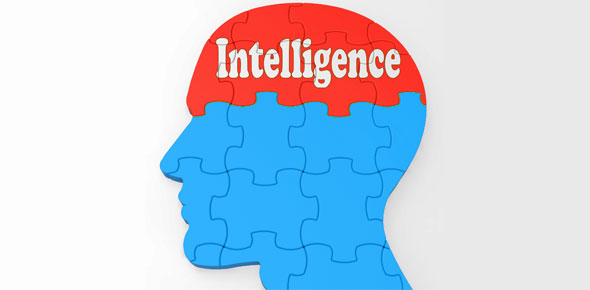# IQ Quiz For Math Geeks

Approved & Edited by ProProfs Editorial Team
The editorial team at ProProfs Quizzes consists of a select group of subject experts, trivia writers, and quiz masters who have authored over 10,000 quizzes taken by more than 100 million users. This team includes our in-house seasoned quiz moderators and subject matter experts. Our editorial experts, spread across the world, are rigorously trained using our comprehensive guidelines to ensure that you receive the highest quality quizzes.
| By Apple1142
A
Apple1142
Community Contributor
Quizzes Created: 1 | Total Attempts: 133
Questions: 5 | Attempts: 133SettingsHello whoever is reading this let me tell you how this quiz is i wil test your iq. So good luck.

• 1.

### What is 79 times 47 ?

• A.

3,713

• B.

250,000

• C.

I really don't care

• D.

8

• E.

7947

A. 3,713
Explanation
The correct answer is obtained by multiplying 79 and 47 together, which equals 3,713.

Rate this question:

• 2.

### If 5 times 20 = 100 what is 5 times 2490 =

12450
Explanation
The given question states that 5 times 20 equals 100. Therefore, if we multiply 5 by any number, we can find the answer by multiplying that number by 20 and then dividing it by 100. In this case, 5 times 2490 would be 2490 multiplied by 20, which equals 49800. Finally, dividing 49800 by 100 gives us the answer of 498. Thus, the correct answer is 12450.

Rate this question:

• 3.

• 4.

### What is the square root of 144

• A.

Who cares

• B.

Idk 144

• C.

12

• D.

7

• E.

123

C. 12
Explanation
The square root of a number is a value that, when multiplied by itself, gives the original number. In this case, the square root of 144 is 12 because 12 multiplied by itself equals 144.

Rate this question:

• 5.

### What is the square root of 6

• A.

2

• B.

3

• C.

4

• D.

5

• E.

Both a and b

E. Both a and b
Explanation
The square root of 6 is a number that, when multiplied by itself, equals 6. Both 2 and 3 are valid square roots of 6 because 2 multiplied by 2 equals 4, and 3 multiplied by 3 equals 9. Therefore, both options a and b are correct answers.

Rate this question:

Related TopicsBack to top